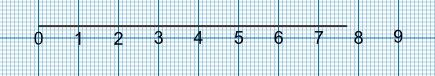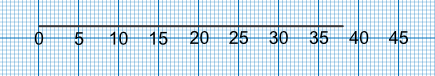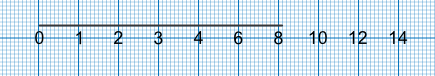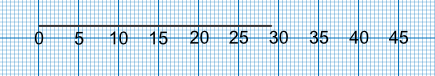Graphs and Scales

## Graphs and Scales

Scales are used to indicate values on graphs. The distance between successive values must be regular.

Check the scale to see by what value the scale is increasing: it may go up in 1s, 2s, 5s, 10s or some other increment. Use the lighter lines to determine intermediate values.

The example below shows a scale which increases in single values. The line stops between 7 and 8. It is seven faint lines after the value of 7 which gives it a value of 7 + 0.7 = 7.7.The next example shows the scale going up in increments of 5. The line is 6 faint lines past the value of 35. Each of the 2 faint lines is worth 1, so 6 lines is worth 3. The value of the line is 35 + 3 = 38.## Example 1

What is wrong with this scale?The scale increases with a value of 1 until it reaches 8, then it increases with a value of 2. The scale is not consistent.

Answer: The scale is not consistent.

## Example 2

What is the value of the line?The scale increases in 5s, each set of 2 lines is worth 1. It is 2 lines short of 30, therefore 29.